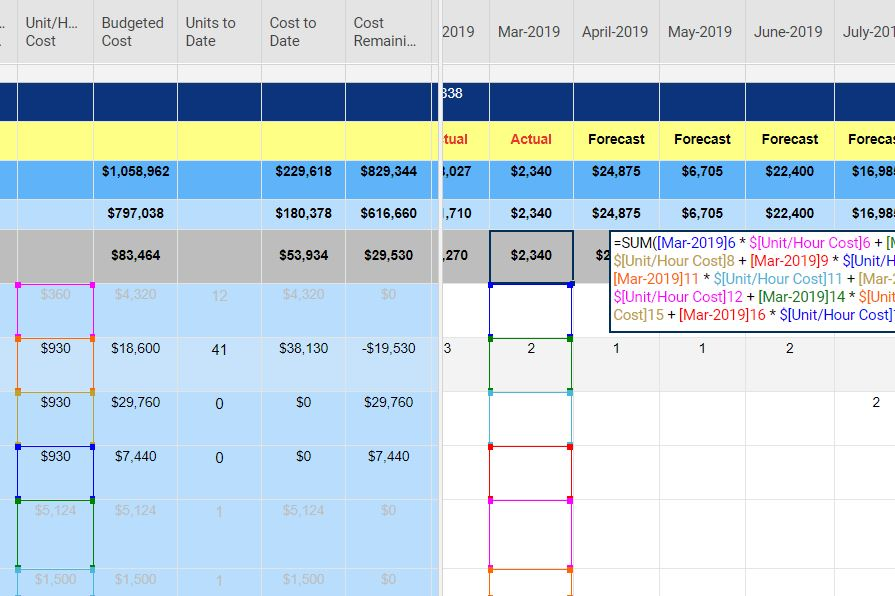# Multiply across columns, then sum children

I would like to use the sum children formula, however the calculation includes other columns before it can sum.

The month column contains number of units, while the cost of each unit is in another column.  The sum for the month = #units * cost per unit.  Can the children formula be used so that the formula doesn't have to be edited every time a new row is added?